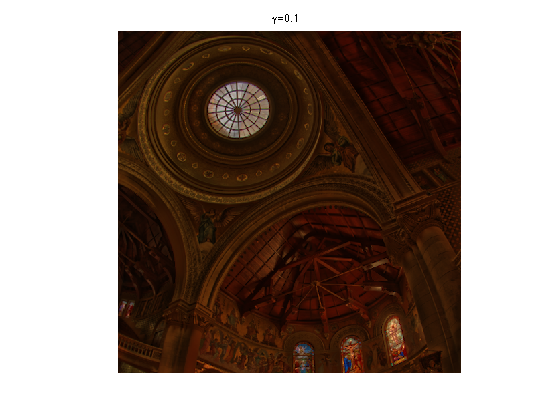$\newcommand{\NN}{\mathbb{N}} \newcommand{\CC}{\mathbb{C}} \newcommand{\GG}{\mathbb{G}} \newcommand{\LL}{\mathbb{L}} \newcommand{\PP}{\mathbb{P}} \newcommand{\QQ}{\mathbb{Q}} \newcommand{\RR}{\mathbb{R}} \newcommand{\VV}{\mathbb{V}} \newcommand{\ZZ}{\mathbb{Z}} \newcommand{\FF}{\mathbb{F}} \newcommand{\KK}{\mathbb{K}} \newcommand{\UU}{\mathbb{U}} \newcommand{\EE}{\mathbb{E}} \newcommand{\Aa}{\mathcal{A}} \newcommand{\Bb}{\mathcal{B}} \newcommand{\Cc}{\mathcal{C}} \newcommand{\Dd}{\mathcal{D}} \newcommand{\Ee}{\mathcal{E}} \newcommand{\Ff}{\mathcal{F}} \newcommand{\Gg}{\mathcal{G}} \newcommand{\Hh}{\mathcal{H}} \newcommand{\Ii}{\mathcal{I}} \newcommand{\Jj}{\mathcal{J}} \newcommand{\Kk}{\mathcal{K}} \newcommand{\Ll}{\mathcal{L}} \newcommand{\Mm}{\mathcal{M}} \newcommand{\Nn}{\mathcal{N}} \newcommand{\Oo}{\mathcal{O}} \newcommand{\Pp}{\mathcal{P}} \newcommand{\Qq}{\mathcal{Q}} \newcommand{\Rr}{\mathcal{R}} \newcommand{\Ss}{\mathcal{S}} \newcommand{\Tt}{\mathcal{T}} \newcommand{\Uu}{\mathcal{U}} \newcommand{\Vv}{\mathcal{V}} \newcommand{\Ww}{\mathcal{W}} \newcommand{\Xx}{\mathcal{X}} \newcommand{\Yy}{\mathcal{Y}} \newcommand{\Zz}{\mathcal{Z}} \newcommand{\al}{\alpha} \newcommand{\la}{\lambda} \newcommand{\ga}{\gamma} \newcommand{\Ga}{\Gamma} \newcommand{\La}{\Lambda} \newcommand{\Si}{\Sigma} \newcommand{\si}{\sigma} \newcommand{\be}{\beta} \newcommand{\de}{\delta} \newcommand{\De}{\Delta} \renewcommand{\phi}{\varphi} \renewcommand{\th}{\theta} \newcommand{\om}{\omega} \newcommand{\Om}{\Omega} \renewcommand{\epsilon}{\varepsilon} \newcommand{\Calpha}{\mathrm{C}^\al} \newcommand{\Cbeta}{\mathrm{C}^\be} \newcommand{\Cal}{\text{C}^\al} \newcommand{\Cdeux}{\text{C}^{2}} \newcommand{\Cun}{\text{C}^{1}} \newcommand{\Calt}{\text{C}^{#1}} \newcommand{\lun}{\ell^1} \newcommand{\ldeux}{\ell^2} \newcommand{\linf}{\ell^\infty} \newcommand{\ldeuxj}{{\ldeux_j}} \newcommand{\Lun}{\text{\upshape L}^1} \newcommand{\Ldeux}{\text{\upshape L}^2} \newcommand{\Lp}{\text{\upshape L}^p} \newcommand{\Lq}{\text{\upshape L}^q} \newcommand{\Linf}{\text{\upshape L}^\infty} \newcommand{\lzero}{\ell^0} \newcommand{\lp}{\ell^p} \renewcommand{\d}{\ins{d}} \newcommand{\Grad}{\text{Grad}} \newcommand{\grad}{\text{grad}} \renewcommand{\div}{\text{div}} \newcommand{\diag}{\text{diag}} \newcommand{\pd}{ \frac{ \partial #1}{\partial #2} } \newcommand{\pdd}{ \frac{ \partial^2 #1}{\partial #2^2} } \newcommand{\dotp}{\langle #1,\,#2\rangle} \newcommand{\norm}{|\!| #1 |\!|} \newcommand{\normi}{\norm{#1}_{\infty}} \newcommand{\normu}{\norm{#1}_{1}} \newcommand{\normz}{\norm{#1}_{0}} \newcommand{\abs}{\vert #1 \vert} \newcommand{\argmin}{\text{argmin}} \newcommand{\argmax}{\text{argmax}} \newcommand{\uargmin}{\underset{#1}{\argmin}\;} \newcommand{\uargmax}{\underset{#1}{\argmax}\;} \newcommand{\umin}{\underset{#1}{\min}\;} \newcommand{\umax}{\underset{#1}{\max}\;} \newcommand{\pa}{\left( #1 \right)} \newcommand{\choice}{ \left\{ \begin{array}{l} #1 \end{array} \right. } \newcommand{\enscond}{ \left\{ #1 \;:\; #2 \right\} } \newcommand{\qandq}{ \quad \text{and} \quad } \newcommand{\qqandqq}{ \qquad \text{and} \qquad } \newcommand{\qifq}{ \quad \text{if} \quad } \newcommand{\qqifqq}{ \qquad \text{if} \qquad } \newcommand{\qwhereq}{ \quad \text{where} \quad } \newcommand{\qqwhereqq}{ \qquad \text{where} \qquad } \newcommand{\qwithq}{ \quad \text{with} \quad } \newcommand{\qqwithqq}{ \qquad \text{with} \qquad } \newcommand{\qforq}{ \quad \text{for} \quad } \newcommand{\qqforqq}{ \qquad \text{for} \qquad } \newcommand{\qqsinceqq}{ \qquad \text{since} \qquad } \newcommand{\qsinceq}{ \quad \text{since} \quad } \newcommand{\qarrq}{\quad\Longrightarrow\quad} \newcommand{\qqarrqq}{\quad\Longrightarrow\quad} \newcommand{\qiffq}{\quad\Longleftrightarrow\quad} \newcommand{\qqiffqq}{\qquad\Longleftrightarrow\qquad} \newcommand{\qsubjq}{ \quad \text{subject to} \quad } \newcommand{\qqsubjqq}{ \qquad \text{subject to} \qquad }$

Bilateral Filtering

# Bilateral Filtering

This tour explores edge preserving filtering and in particular the bilateral filter, with applications to denoising and detail enhancement.

## Installing toolboxes and setting up the path.

You need to download the following files: signal toolbox and general toolbox.

You need to unzip these toolboxes in your working directory, so that you have toolbox_signal and toolbox_general in your directory.

For Scilab user: you must replace the Matlab comment '%' by its Scilab counterpart '//'.

Recommandation: You should create a text file named for instance numericaltour.sce (in Scilab) or numericaltour.m (in Matlab) to write all the Scilab/Matlab command you want to execute. Then, simply run exec('numericaltour.sce'); (in Scilab) or numericaltour; (in Matlab) to run the commands.

Execute this line only if you are using Matlab.

getd = @(p)path(p,path); % scilab users must *not* execute this


Then you can add the toolboxes to the path.

getd('toolbox_signal/');
getd('toolbox_general/');


## Gaussian Linear Filtering

The most basic filtering operation is the Gaussian filtering, that tends to blur edges.

Image size.

n = 256*2;


name = 'hibiscus';
f0 = rescale(crop( sum(f0,3) ,n));


A Gaussian filter of variance $$\si$$ reads $\Gg_\si(f)(x) = \frac{1}{Z} \sum_y G_\si(x-y) f(y) \qwhereq Z = \sum_y G_\si(y),$ and where the Gaussian kernel is defined as: $G_\si(x) = e^{-\frac{\norm{x}^2}{2\si^2}}.$

A convolution can be computed either over the spacial domain in $$O(N\si_x^2)$$ operations or over the Fourier domain in $$O(N \log(N))$$ operations. Depending on the value of $$\si_x$$, one should prefer either Fourier or spacial domain. For simplicity, we consider here the Fourier domain (and hence periodic boundary conditions).

Define a Gaussian function, centered at the top left corner (because it corresponds to the 0 point for the FFT).

x = [0:n/2-1, -n/2:-1];
[Y,X] = meshgrid(x,x);
GaussianFilt = @(s)exp( (-X.^2-Y.^2)/(2*s^2) );


Display a recentered example of Gaussian.

clf;
imageplot(fftshift(GaussianFilt(40)));Define a shortcut to perform linear Gaussian filtering over the Fourier domain. This function is able to process in parallel a 3D block F by filtering each F(:,:,i).

Filter = @(F,s)real( ifft2( fft2(F).*repmat( fft2(GaussianFilt(s)), [1 1 size(F,3)] ) ) );


Example of filtering $$\Gg_\si(f_0)$$.

clf;
imageplot( Filter(f0,5) );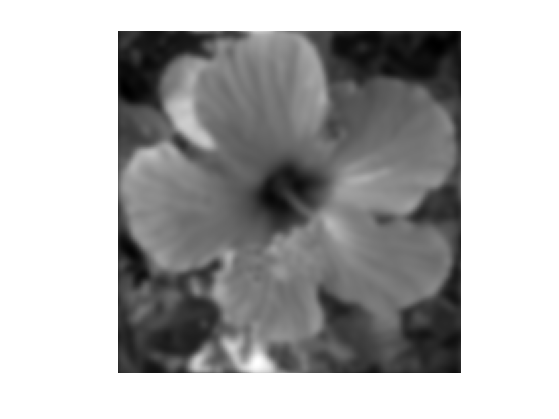Exercice 1: (check the solution) Display a filtering $$\Gg_\si(f_0)$$ with increasing size $$\si$$.

exo1;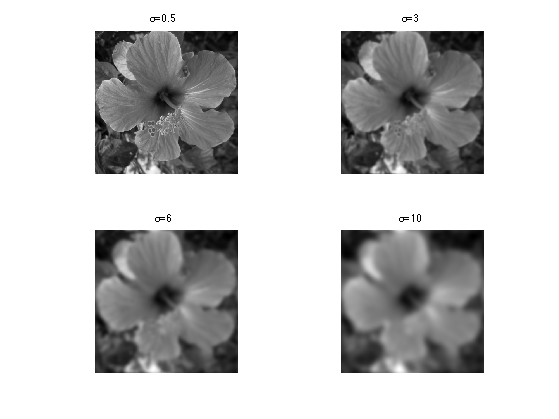## Bilateral Filter

The bilateral filter is a spacially varying filter that better preserves edges than the Gaussian filter.

It was first introduced in:

Carlo Tomasi and Roberto Manduchi, Bilateral Filtering for Gray and Color Images, Proceedings of the ICCV 1998

A very good set of ressources concerning the bilateral filter can be found on Sylvain Paris web page. It includes a fast implementation, research and review papers.

Given a spacial width $$\si_x$$ and a value width $$\si_v$$, the filter opterates as: $\Bb_{\si_x,\si_v}(f)(x) = \frac{1}{Z_x} \sum_y G_{\si_x}( x-y ) G_{\si_v}(f(x)-f(y)) f(y)$ where the normalizing constant is $Z_x = \sum_y G_{\si_x}( x-y ) G_{\si_v}(f(x)-f(y)).$

At a given pixel $$x$$, it corresponds to an averaring with the data-dependant kernel $$G_{\si_x}( x-y ) G_{\si_v}(f(x)-f(y))$$.

## Bilateral Filter by Stacking

Implementing the bilateral filter directly over the spacial domain requires $$O( N \si_x^2 )$$ operations where $$N$$ is the number of pixels.

A fast approximate implementation is proposed in:

Fast Bilateral Filtering for the Display of High-Dynamic-Range Images, Fredo Durand and Julie Dorsey, SIGGRAPH 2002.

It exploits the fact that for all pixels $$x$$ with the same value $$v=f(x)$$, the bilateral filter can be written as a ratio of convolution $\Bb_{\si_x,\si_v}(f)(x) = F_v(x) = \frac{ [G_{\si_x} \star ( f \cdot W_v )](x) }{ [G_{\si_x} \star W_v](x) }$ where the weight map reads $W_v(x) = G_{\si_v}(v-f(x)).$

Instead of computing all possible weight maps $$W_v$$ for all possible pixel values $$v$$, one considers a subset $$\{v_i\}_{i=1}^p$$ of $$p$$ values and computes the weights $$\{ W_{v_i} \}_i$$.

Using these convolutions, one thus optains the maps $$\{ F_{v_i} \}_i$$, that are combined to obtain an approximation of $$\Bb_{\si_x,\si_v}(f)$$.

The computation time of the method is $$O(p N\log(N))$$ over the Fourier domain (the method implemented in this tour) and $$O(p N \si_x^2)$$ over the spacial domain.

Value of $$\sigma_x$$:

sx = 5;


Value of $$\sigma_v$$:

sv = .2;


Number $$p$$ of stacks. The complexity of the algorithm is proportional to the number of stacks.

p = 10;


Function to build the weight stack $$\{ W_{v_i} \}_i$$ for $$v_i$$ uniformly distributed in $$[0,1]$$.

Gaussian = @(x,sigma)exp( -x.^2 / (2*sigma^2) );
WeightMap = @(f0,sv)Gaussian( repmat(f0, [1 1 p]) - repmat( reshape((0:p-1)/(p-1), [1 1 p]) , [n n 1]), sv );


Compute the weight stack map. Each W(:,:,i) is the map $$W_{v_i}$$ associated to the pixel value $$v_i$$.

W = WeightMap(f0,sv);


Exercice 2: (check the solution) Display several weights $$W_{v_i}$$.

exo2;Shortcut to compute the bilateral stack $$\{ F_{v_i} \}_i$$.

bileteral_stack_tmp = @(f0,sx,W)Filter(W.*repmat(f0, [1 1 p]), sx) ./ Filter(W, sx);
bileteral_stack = @(f0,sx,sv)bileteral_stack_tmp(f0,sx,WeightMap(f0,sv));


Compute the bilateral stack $$\{ F_{v_i} \}_i$$.

F = bileteral_stack(f0,sx,sv);


Exercice 3: (check the solution) Display several stacks $$F_{v_i}$$.

exo3;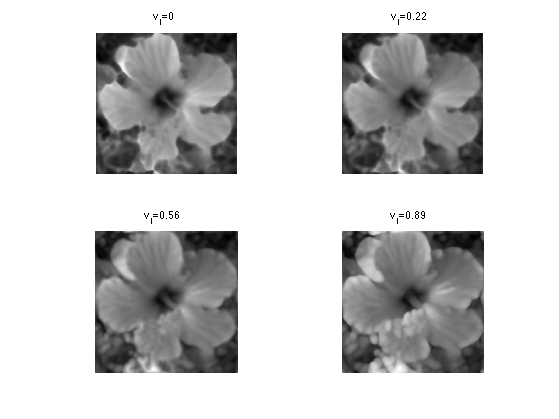Destacking corresponds to selecting a layer $$I(x) \in \{1,\ldots,p\}$$ at each pixel. $f_I(x) = F_{ v_{I(x)} }(x).$

Shortcut for de-stacking using a set of indexes.

[y,x] = meshgrid(1:n,1:n);
indexing = @(F,I)F(I);
destacking = @(F,I)indexing(F,x + (y-1)*n + (I-1)*n^2);


The simplest reconstruction method performs the destacking using the nearest neighbor value: $I(x) = \uargmin{ 1 \leq i \leq p } \abs{f(x)-v_i}.$

Shortcut for performing de-stacking by nearest neighbor interpolation.

bilateral_nn = @(f0,sx,sv)destacking( bileteral_stack(f0,sx,sv), round( f0*(p-1) ) + 1 );


Display.

fNN = bilateral_nn(f0,sx,sv);
clf;
imageplot( fNN );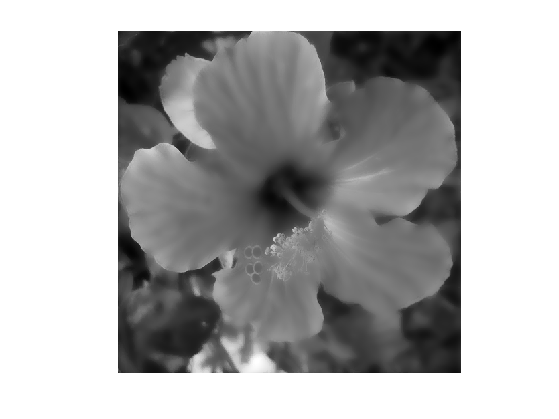A better reconstruction is obtained by using a first order linear interpolation to perform the destacking. $\Bb_{\si_x,\si_v}(f)(x) \approx (1-\la(x))f_{I(x)}(x) + \la(x) f_{I(x)+1}(x)$ where $$I(x)$$ and $$\la(x)$$ are defined as $f(x) = (1-\la(x)) v_{I(x)} + \la(x) v_{I(x)+1} \qwhereq \la(x) \in [0,1).$

Shortcut for the linear interpolation reconstruction.

frac = @(x)x-floor(x);
lininterp = @(f1,f2,Fr)f1.*(1-Fr) + f2.*Fr;
bilateral_lin1 = @(F,f0)lininterp( destacking(F, clamp(floor(f0*(p-1)) + 1,1,p) ), ...
destacking(F, clamp(ceil(f0*(p-1)) + 1,1,p) ), ...
frac(f0*(p-1)) );
bilateral_lin = @(f0,sx,sv)bilateral_lin1(bileteral_stack(f0,sx,sv), f0);


Exercice 4: (check the solution) Compare nearest-neighbor and linear destacking.

exo4;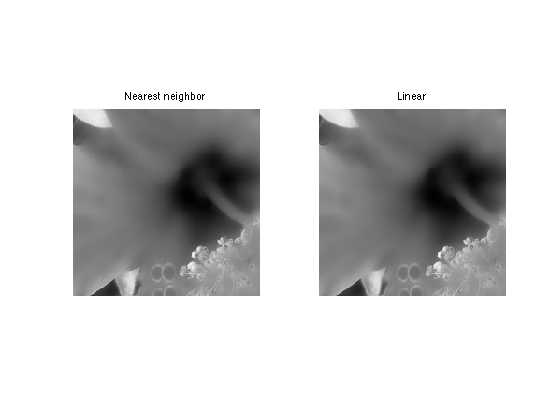Exercice 5: (check the solution) Study the influence of $$\sigma_x$$ on the filter, for a fixed $$\sigma_v=0.2$$.

exo5;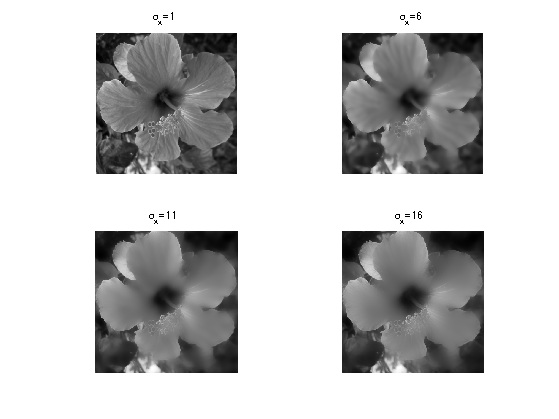Exercice 6: (check the solution) Study the influence of $$\sigma_v$$ on the filter, for a fixed $$\sigma_x=8$$.

exo6;Note that this stack implementation of the bilateral filter can be quite inacurate, in particular if $$p$$ is small. A more precise fast implementation is described in:

A Fast Approximation of the Bilateral Filter using a Signal Processing Approach, Sylvain Paris and Frédo Durand, International Journal of Computer Vision (IJCV'09)

## Denoising using the Bilateral Filter

The first basic application of the bilateral filter is for denoising. It performs a filtering that respects edges.

Noise level.

mu = .05;


Create a noisy image $$f = f_0 + w$$ where $$w$$ is a Gaussian white noise of variance $$\mu^2$$.

f = f0 + randn(n,n)*mu;


Display the noisy image.

clf;
imageplot(clamp(f));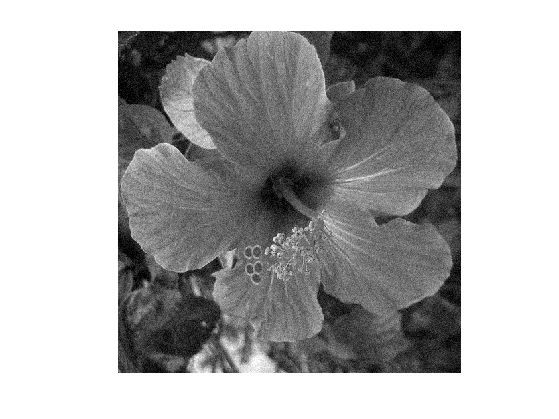Perform denoising using the bilateral filter.

sx = 4; sv = .2;
clf;
imageplot( clamp(bilateral_lin(f,sx,sv)) );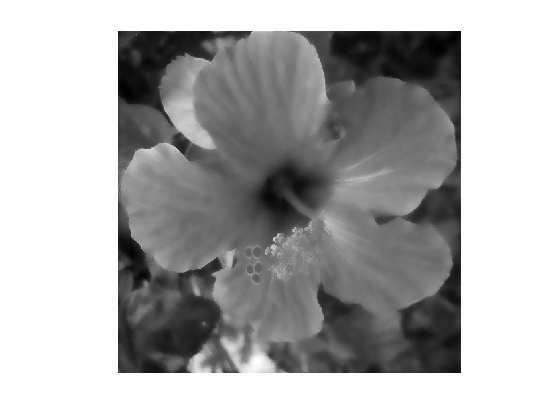Exercice 7: (check the solution) Compute the optimal parameter $$(\sigma_x,\sigma_v)$$ to maximize the SNR between $$f_0$$ and the filtered image. Record the optimal denoising result in fOpt.

exo7;


Display optimal denoising.

clf;
imageplot(clamp(fOpt), ['Bilateral, SNR=' num2str(snr(f0,fOpt),3) 'dB']);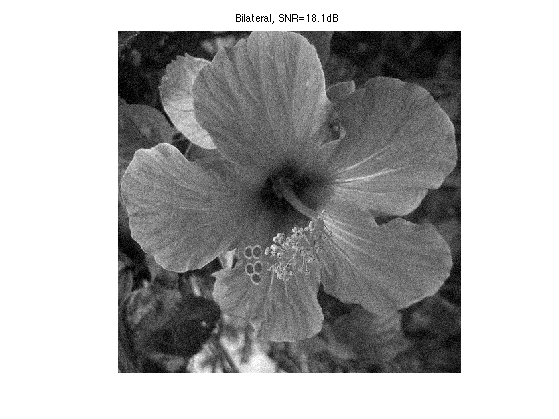Exercice 8: (check the solution) Compare with translation invariant wavelet thresholding.

exo8;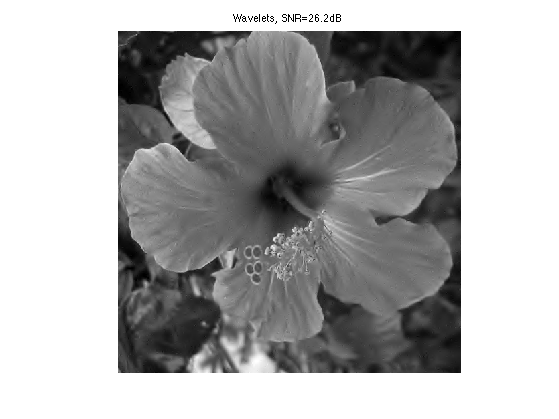## Detail Enhancement

The bilateral filters is able to remove fine scale details (noise, textures) and retain only the cartoon content of the image (shape edges).

Set up parameters $$\si_x,\si_v$$.

sx = 4;
sv = .2;


Performm the filtering to obtain the base layer $$f_1 = \Bb_{\si_x,\si_v}(f_0)$$.

f1 = bilateral_lin(f0,sx,sv);


Compute the detail layer $$r = f_0 - f_1$$.

r = f0 - f1;


Display the base layer.

clf;
imageplot(f1);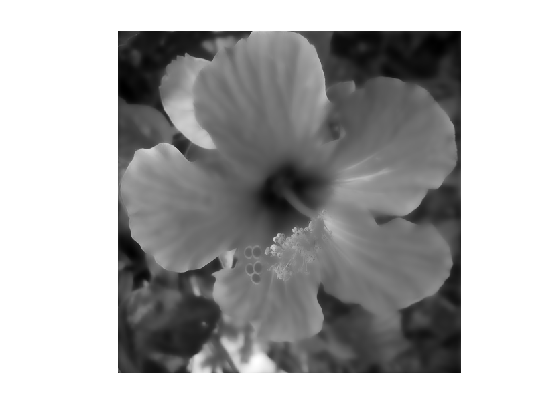Display the residual.

clf;
imageplot(r);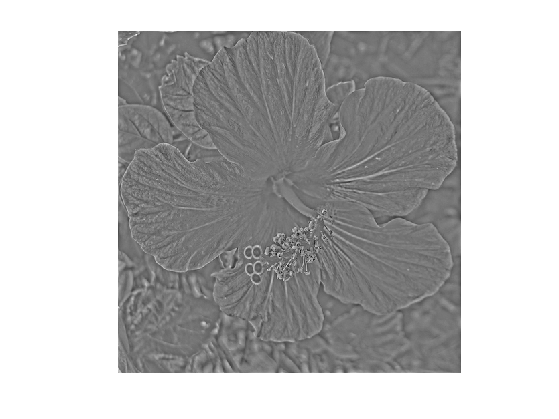Exercice 9: (check the solution) Perform detail boosting by enhancing the detail layer. For instance use various non-linear remapping of the intensities such as $$f_1 + \ga r^\al$$ for some values of $$\ga \geq 1$$ and $$\al>0$$.

exo9;Exercice 10: (check the solution) Extend the bilateral filter for color images.

exo10;


## High Dynamic Range Tone Mapping

Tone mapping corresponds to compressing the contrast of an image that contains a large dynamic range, in order to be viewable on a low dynamic range display.

Load a high dynamic range image.

addpath('toolbox_additional/');
name = 'memorial';
p = min(size(f,1),size(f,2));
f = rescale( f(1:p,1:p,:) );
f = rescale( clamp( image_resize(f,n,n) ) );


The pixel values can take very large value and leads to over exposition (saturation) if displayed with a limited range (clamping of the values).

clf;
imageplot(min(f,1e-4));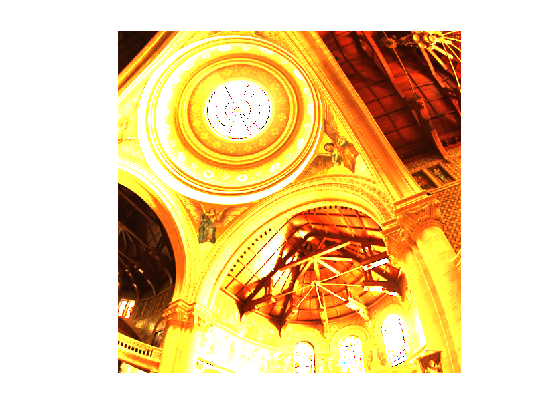Selecting a higher threshold leads to less saturation but more region being under exposed (dark).

clf;
imageplot(min(f,1e-3));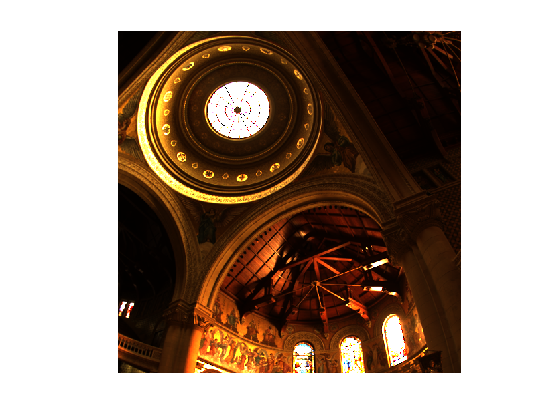A simple way to process a color image is to perform a change of color space and to process only the intensity channel.

For instance one can use a HSV color space and process the intensity channel $$f_V$$.

fhsv = rgb2hsv(f);
fV = fhsv(:,:,3);


Shortcut the reconstruct the image using a modified V channel.

color_recompose = @(fV)hsv2rgb( cat(3, fhsv(:,:,1:2), rescale(fV) ) );

% color_recompose = @(fV1)f.*repmat(fV1./fV, [1 1 3]);


Global tone mapping corresponds to applying a fixed non-linear mapping $$\phi$$ to all the pixel to compress the range by displaying $$\phi(f_V)$$.

A polpular contrast modification is the $$\gamma$$ correction, that uses $$\phi(t) = (t+\epsilon)^\al$$ for a small $$\epsilon>0$$ and $$\al \in (0,1]$$.

epsilon = 1e-5;
alpha = 0.1;
imageplot( color_recompose( (fV+epsilon).^alpha ) );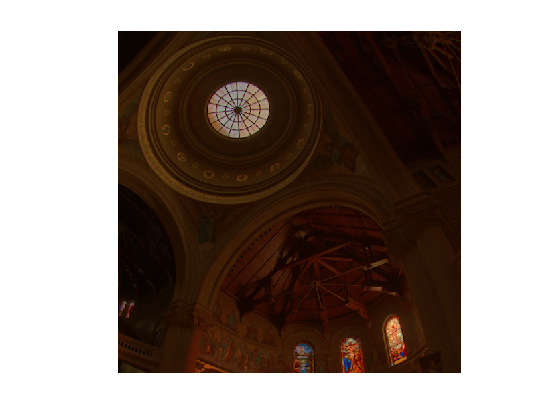Exercice 11: (check the solution) Try several tone mapping operators, such as for instance $\phi_1(t)=\frac{t}{t+\epsilon} \qandq \phi_2(t) = \log(t+\epsilon)$

exo11;Global tone mapping tends to destroy fine scale details. A local tone mapping operator decompose the logarithm $F_V = \frac{ \log(f_V + \epsilon)-a }{ b-a }$ of $$f_V$$ into a base cartoon layer and a textured layer, and only compress the base layer. Here $$a$$ and $$b$$ are compute to ensure that $$F_V \in [0,1]$$.

Compute the rescaled logarithm layer $$F_V$$.

epsilon = 1e-5;
FV = log(fV+epsilon);
a = min(FV(:)); b = max(FV(:));
FV = (FV-a)/(b-a);


The base layer is defined as $$B_V = \Bb_{\si_x,\si_v}(F_V)$$.

sx = 5; sv = .02;
FV1 = bilateral_lin(FV, sx,sv);


The tone mapped intensity image is defined as $\tilde f_V = e^{ ( \ga B_V + F_V - B_V )(b-a)+a } - \epsilon$ where $$\ga \in (0,1]$$ is the compression factor. For $$\ga=1$$, one has $$\tilde f_V = f_V$$.

Display the filtered layer over the log domain.

clf;
imageplot(FV1);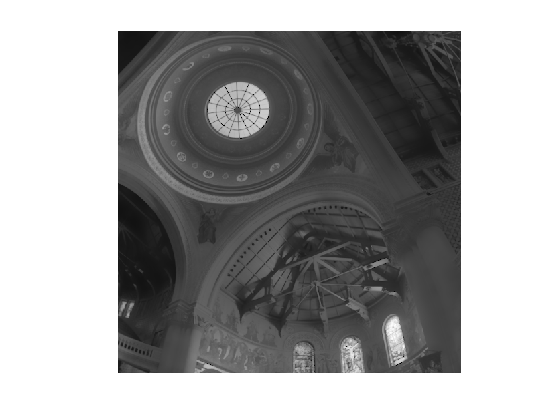Display the residual over the log domain.

clf;
imageplot(FV-FV1);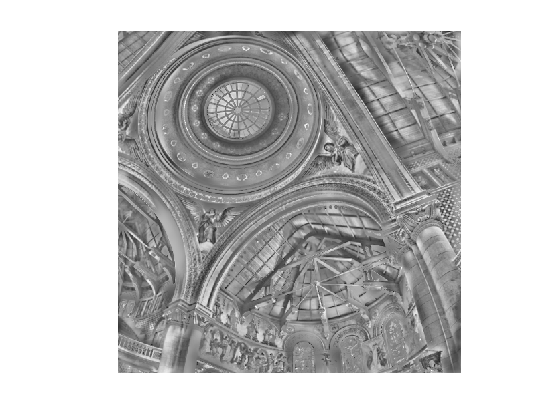Exercice 12: (check the solution) Compute the tone mapped image using $$\tilde f_V$$. Test with several value of $$\ga,\epsilon, \si_x,\si_v$$.

exo12;